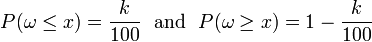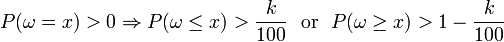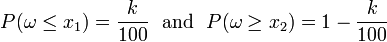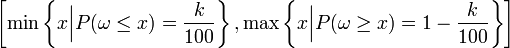# Percentile

Percentiles are statistical parameters which describe the distribution of a (real) value in a population (or a sample). Roughly speaking, the k-th percentile separates the smallest k percent of values from the largest (100-k) percent.

Special percentiles are the median (50th percentile), the quartiles (25th and 75th percentile), the quintiles (20th, 40th, 60th and 80th percentile), and the deciles (the k-th decile is the (10k)-th percentile). Percentiles are special cases of quantiles: The k-th percentile is the same as the (k/100)-quantile.

## Definition

The value x is k-th percentile (for a given k = 1,2,...,99) if$P(\omega\le x) \ge {k\over100} \textrm{\ \ and \ \ } P(\omega\ge x) \ge 1-{k\over100} \, \textrm{.}$

In this definition, P is a probability distribution on the real numbers. It may be obtained either

• from a (theoretical) probability measure (such as the normal or Poisson distribution), or
• from a finite population where it expresses the probability of a random element to have the property,
i.e., it is the relative frequency of elements with this property (number of elements with the property divided by the size of the population),or
• from a sample of size N where it also is the relative frequency which is used to estimate the corresponding percentile for the population from which it was taken.

## Special cases

For most standard continuous distributions (like the normal distribution) the k-th percentile x is uniquely determined by$P(\omega\le x) = {k\over100} \textrm{\ \ and \ \ } P(\omega\ge x) = 1-{k\over100}$

In the general case (e.g., for discrete distributions, or for finite samples) it may happen that the separating value has positive probability:$P(\omega = x) > 0 \Rightarrow P(\omega\le x) > {k\over100} \textrm{\ \ or \ \ } P(\omega\ge x) > 1-{k\over100}$

or that there is a gap in the range of the variable such that, for two distinct x1 < x2, equality holds:$P(\omega\le x_1) = {k\over100} \textrm{\ \ and \ \ } P(\omega\ge x_2) = 1-{k\over100}$

Then every value in the (closed) interval between the smallest and the largest such value$\left [ \min \left\{ x \Bigl\vert P(\omega\le x) = {k\over100} \right\}, \max \left\{ x \Bigl\vert P(\omega\ge x) = 1-{k\over100} \right\} \right]$

is a k-th percentile.

## Examples

The following examples illustrate this:

• Take a sample of 101 values, ordered according to their size:$x_1 \le x_2 \le \dots \le x_{100} \le x_{101}$.
Then the unique k-th percentile is xk + 1.
• If there are only 100 values$x_1 \le x_2 \le \dots \le x_{99} \le x_{100}$.
Then any value between xk and xk + 1 is a k-th percentile.

Example from the praxis:
Educational institutions (i.e. universities, schools...) frequently report admission test scores in terms of percentiles. For instance, assume that a candidate obtained 85 on her verbal test. The question is: How did this student compared to all other students? If she is told that her score correspond to the 80th percentile, we know that approximately 80% of the other candidates scored lower than she and that approximately 20% of the students had a higher score than she had.Some content on this page may previously have appeared on Citizendium.